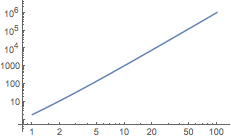Get Started »

# Exponentials & Logarithms

The Wolfram Language represents the exponential constant as E.

Log gives the natural logarithm of an expression:

 In:=⨯ `Log[E^2]`
 Out=Calculate the log base 2:

 In:=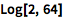⨯ `Log[2, 64]`
 Out=Make plots on a logarithmic scale:

 In:=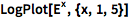⨯ `LogPlot[E^x, {x, 1, 5}]`
 Out=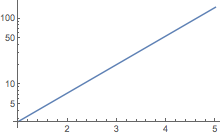Make both axes logarithmic:

 In:=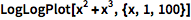⨯ `LogLogPlot[x^2 + x^3, {x, 1, 100}]`
 Out=